﻿ A Comparative Analysis of Heuristic Metaheuristic and Exact Approach to Minimize Make Span of Permutation Flow Shop SchedulingPublications are Open
Access in this journal
Article Versions
Export Article
• Normal Style
• MLA Style
• APA Style
• Chicago Style
Research Article
Open Access Peer-reviewed

### A Comparative Analysis of Heuristic Metaheuristic and Exact Approach to Minimize Make Span of Permutation Flow Shop Scheduling

Tanzila Azad , Asif Ahmed Sarja
American Journal of Industrial Engineering. 2021, 8(1), 1-8. DOI: 10.12691/ajie-8-1-1
Received May 27, 2021; Revised July 03, 2021; Accepted July 12, 2021

### Abstract

The study of permutation flow shop scheduling issues has proved fascinating. Make span reduction has been explored extensively throughout the years as one of the many factors. If there are more than two machines, finding a schedule that optimizes total completion times for Permutation Flow Shop issues is NP Hard. The well-known Nawaz–Enscore–Ham (NEH) heuristic is acknowledged as the top performing approach for the permutation flow shop scheduling issue till now, according to the make span minimization criteria. For large-scale problems, however, the NEH approach takes a long time to discover an estimated optimum solution. Many researchers have offered heuristics and meta-heuristics as the time it takes to compute grows exponentially in proportion to the size of the problem. For the permutation flow shop scheduling problem, we proposed a genetic algorithm with the criterion of minimizing make span as the criteria. The method's performance is compared to that of the well-known NEH algorithm. Computational experiments on benchmark data sets revealed that the proposed approach produces satisfactory solutions in short computational cycles. Furthermore, it just requires a few user-defined parameters, making it relevant to real-world flow shop scheduling challenges. The findings reveal that, when compared to an exact technique, the proposed genetic algorithm (GA) is capable of rapidly seeking optimal solutions for most small-sized instances. Furthermore, the proposed GA continues to function satisfactorily for medium and large-scale instances and generates solutions in a reasonable period.

### 1. Introduction

The permutation flow shop scheduling problem (PFSP) has received a lot of attention in the literature and has a lot of practical applications in manufacturing and service systems. n tasks are performed on m machines in the same sequence in typical permutation flow shop scheduling issues. The goal is to determine the optimum order in which jobs should be processed. The make span is often specified as the goal function. Other performance parameters used in the literature include flow time, earliness, lateness, tardiness, and so on 1. Pinedo 2 describes the subject in depth and proposes a categorization approach for PFSP extensions and variations. Wagner 3 proposes preliminary approaches and mathematical models for job shop and PFSP solutions. Baker 4, Stafford 5, Wilson 6, and Manne 7 are some of the other scholars that propose mathematical models. PFSP is an NP-hard issue for three or more machines, according to Rinnooy Kan 8. To overcome the challenge, a variety of heuristics and meta-heuristics have been developed. The two basic kinds of PFSP heuristics are constructive and improvement heuristics. Nawaz et al. 9 introduced the NEH algorithm as an example of constructive approaches. Palmer 10 and Campbell et al. 11 have shown various constructive algorithms. The NEH heuristic is considered the best constructive heuristic for solving PFSP by Dong et al. 12 and Li et al. 13.

A case study was conducted by Rizkya et al. 14 at a furniture manufacturing firm. The company used to schedule production utilizing the First Come First Serve (FCFS) scheduling mechanism. To reduce the make span, they used the standard NEH method to decide the production sequence. The results were then compared to the FCFS scheduling scheme. The time necessary to finish all orders (make span) was less with the NEH algorithm than with the FCFS approach, according to the findings of production scheduling using the NEH algorithm.

To improve the scheduling of a permutation flow shop, Baskar 15 suggested seven variations of the NEH method. The variations were created by altering the original partial series. The 120 cases suggested by Taillard were utilized to test the algorithms' validity. Except for two, the jobs were initially ordered in non-increasing order in terms of overall processing time. The average percentage increase in make-up from the known maximum limits was less than 4% in all circumstances, which is better than many other basic heuristics. Simply changing the beginning partial sequence increased the make span in three methods. Other partial sequences, in addition to using the first two tasks as the first partial sequence, gave better outcomes than the original NEH approach. The job insertion approach is the most successful portion of the NEH algorithm, followed by the first arrangement of jobs in their entire processing times in a non-increasing sequence. Three of the seven algorithms evaluated in this research are simpler variations of the NEH with the same degree of complexity, but they generated superior results.

P. Bhatt 16 employed two strategies, NEH and Simulated Annealing (SA), to solve PFSPs singly and in combination. She evaluated the NEH and SA algorithms, as well as the combined algorithm, against the current ones, based on outcome and execution time. Standard benchmarks were used to assess the efficiency of the developed method. The initial comparison revealed that the more iterations performed, the better the result.

Y. Jin et al. 17 improved the NEH algorithm's performance in solving PFSPs. A new priority criterion that accounts for the average and mean absolute variance was also presented to adequately explain the distribution style of processing times. A new tie-breaking rule was also designed to enable efficient task insertion with the purpose of minimizing both make span and machine idle time. Statistical studies revealed that the suggested approach is more efficient than current benchmark heuristics. Calculating two objectives relating the calculation timings of each heuristic required more computing time than the single criteria of make span. The results of the tests utilizing the tie-breaking rule revealed that it outperformed the others. There are significant differences between this rule with the other four NEH tie-breaking rules.

To overcome the problem, meta-heuristic algorithms have been studied. Genetic algorithm (GA), SA 18, tabu search (TS) 19, and ant colony optimization (ACO) 20 are examples of meta-heuristic-based approaches. Also, for improving heuristics, the iterated local search (ILS) approach 21 is an excellent example. ILS is a basic yet effective metaheuristic with methods for avoiding local minima and maxima. The perturbation process, which is the most well-known method for jumping to a new restart place, is one of these processes.

The phrase "genetics" is a biological word. Biologically, the genes of a successful parent produce healthier offspring. The same definition underlies the development of GA. In general, a population of structures to apply GA to a problem defines the problem's solution space, with each structure representing a potential solution to the problem. Each structure has a fitness characteristic connected with it. A specified number of structures are then chosen to constitute the initial generation. Simple genetic operators are applied on the parent structures from the current generation to create the next-generation structures. According to the theory that "successful parents create superior children," a structure with a higher fitness value in the present generation has a better chance of being chosen as a parent. This concept may be used to quickly determine the optimal PFSP sequences.

To solve a ten machine PFSP, Noorul Haq et al. 22 employed a feed-forward back-propagation artificial neural network (ANN) based GA. The trained network was subsequently applied to an issue involving a larger number of jobs. The network was taught to solve tasks in five, six, and optimal sequences with seven tasks. This well-trained network was subsequently applied to a problem involving a larger number of workers. From the random insertion disturbance methodology, the sequence acquired using the neural network was employed to generate the GA starting population (RIPS). The magnitude of the sequence acquired using this approach (ANN-GA-RIPS) was compared to that acquired using GA from a randomly selected population. Five 20-job and 50-job problems were addressed, with the number of machines being constant at 10, and their dependability compared to make span values. It was discovered that the ANN-GA-RIPS approach performed better than ANN-GA when starting with a random population. The results were compared to those produced utilizing Taillard's benchmark problems of NEH's heuristic and upper bounds. The ANN-GA-RIPS heuristic outperformed the NEH heuristic, with the results falling within 5% of the upper boundaries.

To reduce the make span in a permutation flow shop, R. Robert et al. 23 designed an efficient genetic algorithm (EGA). The suggested approach was put to the test using a number of well-known issues from the literature. EGA is a simple and efficient algorithm for solving single and multi-objective problems in the flow shop. The EGA resolution result was compared to current study findings. The results reveal that the anticipated EGA outperforms NPFS-ACO algorithms in finding the flow shop scheduling issue using the make span criteria, with an average improvement of 1.42 percent. The generated EGA was found to be exceptionally abundant, suited for twenty tasks with five machine drawbacks, and yielding seven higher outcomes from ten EGA samples. As a result of this change, two distinct meta-heuristic methods for classifying explicitly real coded genetic algorithm (RCGA) and EGA flow shop planning issues were developed. When compared to RCGA, however, EGA performed admirably.

Widyawati and G Waliadi 24 developed a GA and applied it in a manufacturing firm that makes steam turbine parts for power plants. Because the firm was receiving huge orders, the scheduling procedure was becoming increasingly crucial. To overcome this difficulty, the authors created an algorithm and used it to try to discover the best sequences. After computing the make span using the algorithm's sequence, it was discovered that the make span time with 5 jobs and 6 operations was 53 days, which was a significant improvement over the previous result.

In this paper, the concepts of GAs will be applied to the solutions of n-jobs, m-machines flow shop scheduling problems with make span (Cmax) as the criterion. Then the result will be compared with Taillards instances to check its effectiveness.

### 2. Problem Definition

In this example of a permutation flow shop, N jobs are to be processed consecutively on M machines and the order of jobs on every machine remains the same. The objective is to minimize the make span by finding an optimal sequence. The problem can be categorized as Fm|prmu|Cmax, where F represents an m machine flow shop, prmu stands for permutation, and Cmax, represent make span of the PFSP.

The following assumptions are considered in this study:

1. All jobs are available for processing at time 0.

2. Each job can only be processed on one machine and each machine can process only one job at a time.

3. No preemption is allowed, i.e., once the processing of a job has started, it must be completed without interruption.

4. Only permutation schedules are considered here, i.e., all jobs have the same processing order on all machines.

2.1. Mathematical Formulation

The following notations are applied to formulate the mathematical model and proposed algorithm

Parameters,

CT is the total make span

N is the number of jobs

M is the number of machinesis the index of number of jobs,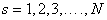is the job to be processed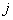represents the machine to process the jobs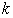represents the position of job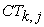is the time of end of processing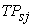is the time of processing by job i in machine j

Decision Variable:1, if task i is in position k

0 otherwise.

The objective function of the model expressed by,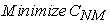(1)

Equation (1) is about finding the best sequence of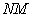to find the lowest make span. However, the objective function is subjected to a few constraints, which are described below.(2)(3)

Equations (2) and (3) represents a discrete optimization problem involving the decision between two alternatives, that is, if a job is processed at a given machine, the other tasks cannot be processed concurrently at the same machine.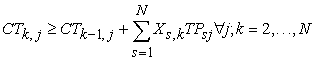(4)

Equation (4) shows that for a scheduled job to be processed in machine j, from the second order of sequencing, it must present a make span greater than or equal to the make span of a task placed earlier on the same machine j, plus the processing time of the same job on resource j.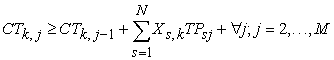(5)

Equation (5) represents that the make span of the job of order k on machine j is greater than or equal to the make span of the same job on machine j-1 plus the sum of the products of the binary variableand the processing times(6)

Equation (5) presents the constraint that the make span of such task is greater than or equal to the make span of the job placed in the sequence 1 of the production scheduling, at machine j-1, plus the sum of the products of the binary variable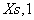and the processing times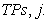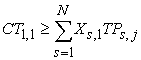(7)

Equation (7) shows that the make span of this task must be greater than or equal to the sum of the products of the binary variable (X1,1, X2,1, X3,1, X4,1) and the processing times (TP1,1, TP2,1, TP3,1, TP4,1).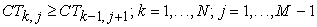(8)

Equation (8) shows that the make span sequenced in the order k on machine j must be greater than or equal to the make span sequenced in the order k-1 on machine j +1, starting from the order of sequence 1 on machine 1.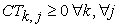(9)

Finally, Equation 9 represents the non-negativity of the model.

### 3. Proposed Genetic Algorithm

As in the GA, the evolutionary portion is carried out using chromosome selection, crossover, and mutation operations as per the following algorithm. The genetic algorithm is depicted in Figure 1 as a flow map, with every chromosome representing a solution determined by a representation mechanism.

• Figure 1. The flowchart of the proposed genetic algorithm

Genetic algorithm:

Start

Step 1: Read problem instance data

Step 2: Set GA parameters

Step 3: Generate first individual by NEH algorithm and Population size (Popsize) -1 randomly an initial population

Step 4: For Generation =1 to Maximum Generation

Step 5: Evaluate fitness of the individuals of population

Step 6: Select parent from the population

Step 7: Apply GA operators (crossover (two-point crossover) and mutation (Swap))

Step 8: Create new population

Step 9: Apply repair process for constraint violation

Step 10: Replace the current with the new generation

Step 11: Stopping Criteria met

Step 12: Otherwise go to step 5

End

### 4. Results and Experimental Analysis

As a result, rather than comparing the proposed GA to any known algorithms, an explicit approach is used. This section is divided into two subsections: parameter standardizations and experimental result and discussions.

4.1. Parameter Standardization

Subsequently the efficiency of a meta(heuristic) is dependent on its parameters (K. Wang et al., 2015), tuning the main parameters of GA, like the Tournament size(Tsize), size of populations (Popsize), generations number (Gen), probability of crossover (Pc), and probability of mutation (Pm) is essential. As compared to the classic full factorial experimental design, Taguchi's design of experiment method (Nair, 1992) significantly decreases the number of experiments expected, and is thus used to standardize the parameters of the proposed GA.

Since there are five parameters, each of which has four stages seen in Table 1 and a total of 45 = 1024 experiments must be required to be performed within the maximum factorial experimental framework. The Taguchi technique, on the other hand, solely examines 16 variations of factor levels based on the orthogonal array L16(45) seen in Table 2. To evaluate an appropriate GA parameter configuration, each combination algorithm is run ten times independently to ensure that the parameters are correct. GA runs ten times with a specific parameter configuration for this set of samples, and the stopping criteria for each run is close to the maximum number of fitness evaluations. After that, objective value is collected as an efficiency indicator. The inclination of every parameter is showed in Figure 2 in accordance with the Taguchi experimental findings. The x-axis in this diagram represents the primary GA parameters that need to be adjusted, while the y-axis represents the average objective value for every level of parameter. The subsequent parameter of GA could offer satisfactory results: Gen = 20, Tournament size = 5, Pc = 0.12, Pm = 0.05 and Popsize =75.

• Figure 2. The value of Objective for each parameter level of GA

Table 3 shows the value of response and rank of every parameter for every stage based on objective values. This table also displays each parameter's delta values, is the gap in between the highest and lowest average values of response for every element. Number of generation (Gen), for example, has a delta value of 13134 (= 14301- 1166) in Table 3. It is critical to recognize that a parameter with a higher delta value is more significant than another. The Number of generation (Gen) is the most critical parameter for the proposed genetic algorithm, according to the delta value in Table 3, while the Popsize is the least important parameter. To check the parameters, twelve instances were chosen, from Taillard’s benchmark data information of permutation flow shops. Wherever the stopping criteria of every run is set to the generation (Gen) number; it will stop once the number of recent generations reaches the maximum generation (Gen) number.

4.2. Result and Discussions

This section describes computational experiments carried out to investigate the performance of the genetic algorithm. The algorithms are coded in Python and run in an i5 PC with 4 GB RAM. For validating the algorithms, out of the 120 numbers of Taillard (1993) benchmark instances 12 have been used. They are grouped into varying sizes having 20, 50, 100, 200 and 500 jobs and 5, 10 and 20 machines. The following figures illustrate the progress of the algorithms. Table 4 represents the make span for different algorithms using Taillard’s problem sets, Table 5, Table 6, Table 7, and Table 8 represents total make span and sequence obtained using GA varying number of jobs of same number of machines which is taken 5 machines as a sample.

• Figure 3. Total make span using CPLEX, NEH & GA for a 20×5 flow shop

4.2.1. Result Analysis

From Table 4 it can be observed that, firstly an exact solution in terms of total make span was calculated using CPLEX. The objective value shows that exact method (CPLEX) generates exactly same solutions as GA which indicates the validation of the proposed GA. But for such complex problem at hand the commercial software CPLEX could not give any feasible solution with the increased number of variables even for a moderate size of instances. The proposed GA, on the other hand, finds high-quality solutions for medium and large-scale problems in a short amount of time. Then, for the same problem set the make span was calculated using NEH. The make span using NEH & GA turned out to be same as like the exact solution. The comparison is illustrated in Figure 3. This indicates the feasibility of this research.

In the next step, make span was calculated for 11 other problem sets using NEH & GA. For small size flow shop problems like 20×5, 20×20 & medium size flow shop problems like 50×5 & 50×20, the make span using GA was much lower than the make span of NEH algorithm. So, it can be said that the job sequence obtained using the GA developed in this research is better than the sequences obtained using NEH for small and medium size flow shop problems. But for large flow shop problems where, 100,200 or 500 jobs are used, the make span increased for GA than the conventional NEH.

In Table 5, Table 6, Table 7 and Table 8 the obtained sequences and the make span using the sequences are represented for the 4 set of flow shop problems. The number of generations, total population, crossover rate, tournament size and mutation rate were the same for all the 12 instances shown in Table 4. As in the PFSP the job sequence is same for all the machines, the sequence for individual machines is not exhibited in the table.

• Figure 4. Convergence of GA

Figure 4 illustrates the convergence of GA. As the number of generations increases the total make span starts to go downward. At a point, the make span stops to go down for few generations, which can be denoted as the local optima. Then again when the number of generations increases more, the make span again starts to go down. At some point the make span totally stops to decrease and get fixed at that point, even if the no of generations keeps increasing. This point indicates the global optima, and this phenomenon is called the convergence of GA. In this figure, for a 20 job 5 machine flow shop the lowest possibly make span found was 1286 min, in 15 generations.

As we know the NEH is the strongest heuristic for solving PFSP till date, the make span for large flow shops could not be minimized using the GA. But we were able to minimize the make span for small and medium size flow shops. Therefore, from the analysis it is found that results obtained applying the proposed approach is more suitable under considered situation.

### 5. Conclusion

The goal of this thesis is to use a metaheuristic strategy to reduce the overall make span for PFSP, which can outperform NEH. Initially, the issues with the existing algorithms were outlined. After that, a comprehensive literature review was conducted by reading PFSP-related articles. A genetic algorithm was written in Python after a mathematical model was developed. The method was put to the test on 12 different Taillard benchmark tasks. The optimal sequence and total make span were then calculated utilizing the GA that had been constructed. The NEH make span was also calculated. To validate the result of proposed genetic algorithm, it was also programmed in CPLEX. The obtained results using GA was then compared with NEH and the exact solution.

The experimental results were pleasing. The comparison showed that the algorithm performed well and can minimize the make span for small and medium size flow shop problems.

### 6. Future Recommendations

Although the outcome of this work is satisfactory, there are several areas where it may be improved in the future. For small and medium-sized flow shops, the GA described in this work was able to reduce make span, but it is not appropriate for large flow shops. A hybrid metaheuristic may be built to handle this problem by combining GA with other metaheuristics such as the Ant Colony method, Simulated Annealing (SA), Variable Neighborhood Search (VNS), and so on. By hybridizing, the best mutation parents can be discovered, and the GA's performance can be improved.

### References

  Chakraborty UK. (2009). Computational intelligence in flow shop and job shop scheduling. Springer Science & Business Media, Berlin. In article View Article  Pinedo M. (2002). Scheduling – theory, algorithms, and systems. Prentice-Hall, Upper Saddle River. In article  Wagner HM. (1959). An integer linear-programming model for machine scheduling. Nav Res Logist Q 6:131-140. In article View Article  Baker KR. (1974) Introduction to sequencing and scheduling. Wiley, New York. In article  Stafford EF. (1988). On the development of a mixed-integer linear programming model for the flowshop sequencing problem. J Oper Res Soc 39: 1163-1174. In article View Article  Wilson JM. (1989). Alternative formulations of a flow-shop scheduling problem. J Oper Res Soc 40: 395-399. In article View Article  Manne AS. (1960). On the job-shop scheduling problem. Oper Res 8: 219-223. In article View Article  Rinnooy Kan AHG. (1976). Machine scheduling problems: classification, complexity, and computations. Nijhoff, The Hague In article  Nawaz M, Enscore EE Jr, Ham I. (1983). A heuristic algorithm for the m-machine, n-job flow shop sequencing problem. OMEGA 11(1): 91-95. In article View Article  Palmer DS. (1965). Sequencing jobs through a multistage process in the minimum total time: a quick method of obtaining a near optimum. Oper Res Q 16: 101-107. In article View Article  Campbell HG, Dudek RA, Smith ML. (1970). A heuristic algorithm for the n job, m machine sequencing problem. Manag Sci 16(10): B630-B637. In article View Article  Dong X, Huang H, Chen P. (2008). An improved NEH-based heuristic for the permutation flowshop problem. Comput Oper Res 35: 3962-3968. In article View Article  Li XP, Wang YX, Wu C. (2004). Heuristic algorithms for large flowshop scheduling problems. In: Proceedings of the 5th world congress on intelligent control and automation, pp 2999-3003. In article  Rizkya, I. et al. (2019). “Nawaz, Enscore, Ham (NEH) Algorithm to Minimization of Makespan in Furniture Company”, IOP Conference Series: Materials Science and Engineering, 505(1). In article View Article  Baskar, A. (2016). “Revisiting the NEH algorithm- the power of job insertion technique for optimizing the makespan in permutation flow shop scheduling”, International Journal of Industrial Engineering Computations, 7(2), pp. 353-366. In article View Article  Bhatt, P. (2019). “Permutation Flow Shop via Simulated Annealing and NEH”, UNLV Theses, Dissertations, Professional Papers, and Capstones. 3575. In article  Liu, W., Jin, Y. and Price, M. (2016). “A new Nawaz–Enscore–Ham-based heuristic for permutation flow-shop problems with bicriteria of makespan and machine idle time”, Engineering Optimization, 48(10), pp. 1808-1822. In article View Article  Osman I, Potts C. (1989). Simulated annealing for permutation flow shop scheduling. OMEGA 17(6):551-557. In article View Article  Grabowski J, Wodecki M. (2004). A very fast tabu search algorithm for the permutation flowshop problem with makespan criterion. Comput Oper Res 31(11):1891-1909. In article View Article  Rajendran C, Ziegler H. (2004). Ant-colony algorithms for permutation flowshop scheduling to minimize makespan/total flowtime of jobs. Eur J Oper Res. 155(2):426-438. In article View Article  Stützle T. (1998). Applying iterated local search to the permutation flowshop problem. Technical report, AIDA-98-04, Intellctics Group, Computer Science Department, Darmstad University of Technology, Darmstad, Germany. In article  Haq, A. N. et al. (2010). “A hybrid neural network-genetic algorithm approach for permutation flow shop scheduling”, International Journal of Production Research, 48(14), pp. 4217-4231. In article View Article  Jeen Robert, R. B. and Rajkumar, R. (2017). “An effective genetic algorithm for flow shop scheduling problems to minimize makespan”, Mechanika, 23(4), pp. 594-603. In article View Article  Widyawati and Waliadi, G. (2020). “Improved Genetic Algorithm for Flow Shop Scheduling Problem at PT. XYZ”, Journal of Physics: Conference Series, 1477(2). In article View ArticleThis work is licensed under a Creative Commons Attribution 4.0 International License. To view a copy of this license, visit http://creativecommons.org/licenses/by/4.0/